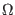Next: Inductors Up: Passive Element Lines Previous: Capacitors   Contents   Index

## Capacitor Model

Type Name: c

The capacitor model contains process information that may be used to compute the capacitance from strictly geometric information.

 name Capacitor Model Parameters parameter units default example m parallel multiplier - 1.0 1.2 cj junction bottom capacitance F/M2 - 5e-5 cjsw junction sidewall capacitance F/M - 2e-11 defw default device width M 1e-6 2e-6 narrow narrowing due to side etching M 0.0 1e-7 tnom parameter measurement temperature C 25 50 tc1 first order temperature coeff./C 0.0 - tc2 second order temperature coeff./C2 0.0 -

The capacitor has a nominal capacitance computed as below, where l and w are parameters from the device line.

C = cj . (l- narrow)(w- narrow) + 2cjsw . (l+ w- 2 . narrow)

After the nominal capacitance is calculated, it is adjusted for temperature by the formula:

C(temp) = C(tnom) . (1 + tc1 . (temp- tnom) + tc2 . (temp- tnom)2 )

Finally, the capacitance is multiplied by the parallel multiplication factor (m).Next: Inductors Up: Passive Element Lines Previous: Capacitors   Contents   Index
Stephen R. Whiteley 2022-09-18